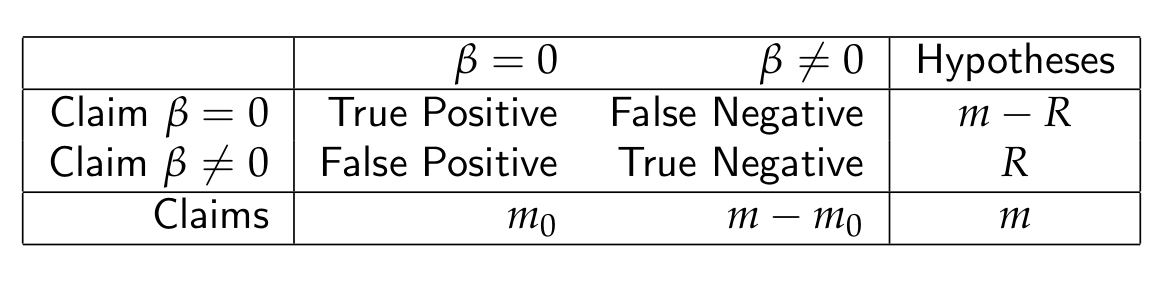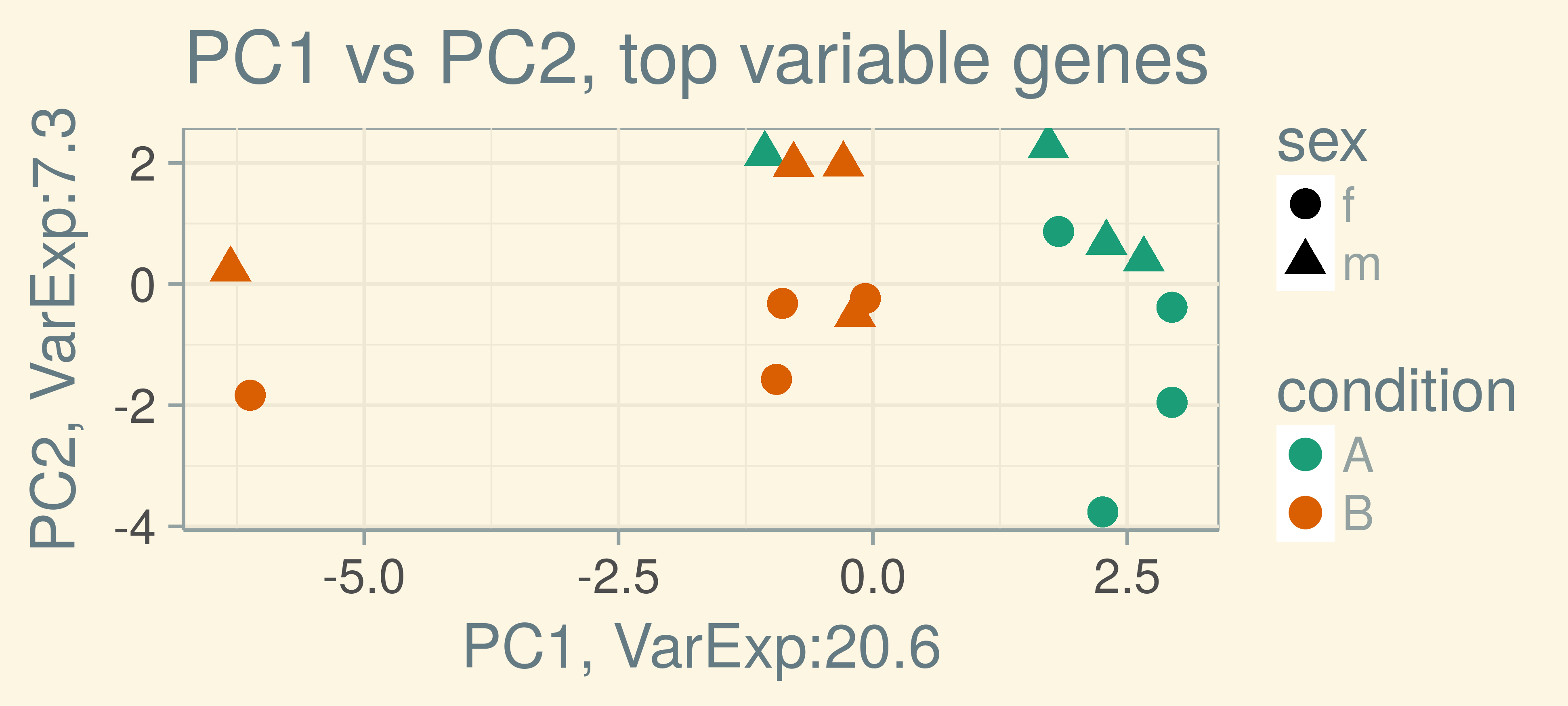# Contents

LAST UPDATE AT

    "Tue Jun 12 09:00:28 2018"

# 1 Required packages and other preparations

library("readxl")
library("BiocStyle")
library("knitr")
library("MASS")
library("RColorBrewer")
library("stringr")
library("pheatmap")
library("matrixStats")
library("purrr")
library("magrittr")
library("entropy")
library("forcats")
library("DESeq2")
library("broom")
library("tidyverse")
library("limma")
library("ggthemes")
library("corpcor")
library("sva")
library("zinbwave")
library("clusterExperiment")
library("clue")
library("sda")
library("crossval")
library("randomForest")
library("keras")

theme_set(theme_solarized(base_size = 18))

data_dir <- file.path("data")

## 1.1 mTEC single cell-RNA-Seq data import

We first import the mTEC data again.

load(file.path(data_dir, "mtec_counts.RData"))
load(file.path(data_dir, "tras.RData"))

## 1.2 Function to compute a PCA

compute_pca <- function(data_mat, ntop = 500, ...){

pvars <- rowVars(data_mat)
select <- order(pvars, decreasing = TRUE)[seq_len(min(ntop,
length(pvars)))]

PCA <- prcomp(t(data_mat)[, select], center = TRUE, scale. = FALSE)
percentVar <- round(100*PCA$sdev^2/sum(PCA$sdev^2),1)

return(list(pca = data.frame(PC1 = PCA$x[,1], PC2 = PCA$x[,2],
PC3 = PCA$x[,3], PC4 = PCA$x[,4], ...),
perc_var = percentVar,
selected_vars = select))

}

# 2 Statistical testing for high–throughput data

In high throughput data we often measure multiple features (e.g. genes) for which we then perform statistical tests (e.g. for differential expression). Choosing a p–value significance cutoff $$\alpha$$ of e.g. 10%, classical testing theory tells us that we will get a false positive result in 10% of the time. This is no longer true if multiple tests are performed simultaneously. At any $$\alpha$$ the probability of making no false rejection goes to zero quite rapidly:

$\underbrace{(1-\alpha)\cdot(1-\alpha)\cdot \dotso \cdot (1-\alpha)}_\text{many--times} \rightarrow 0$

For an $$\alpha$$ of 10% and 50 tests, we get a probability of 0.9^50 = 0.005 to not make a false rejection. So it is clear that we need different error measures for the multiple testing situation. Before we can define them, we need to look at the different error Types.

## 2.1 Error types and error rates

Suppose you are testing a hypothesis that a fold change $$\beta$$ equals zero versus the alternative that it does not equal zero. Let us assume that there are $$m_0$$ non–differentially expressed genes out of $$m$$ genes and that we declare $$R$$ genes as differentially expressed. (i.e. reject the null hypothesis). These are four possible outcomes:• Type I error or false positive — Say that the fold change does not equal zero when it does

• Type II error or false negative — Say that the fold change equals zero when it doesn’t

Just like ordinary significance testing tries to control the false positive rate, there are two types of rates commonly used in multiple testing procedures:

• Family wise error rate (FWER) – The probability of at least one false positive $$\text{Pr}(FP \geq 1)$$

• False discovery rate (FDR) – The rate of false positives among all rejected hypothesis $$E\left[\frac{FP}{FP + TP}\right]$$

The FWER is conceptually closely related to classical significance testing, as it controls the probability of making any false rejection. However, as we will also see below, using a procedure to control it is generally too conservative in large scale problems.

The FDR allows for a certain rate of false positives and is the “typical” error rate controlled in large scale multiple testing problems.

## 2.2 Statistical testing with RNA–Seq data

Consider the following simulated RNA–Seq data, where we have simulated two groups with 8 samples in each group and a 1000 genes. Each group contains 2 batches: males and females. On top of this, there is genetic information on the subjects which we will use later on in the discussion of batch effects and unwanted variation.

The data is stored in an DESeqDataSet, and we apply a variance stabilization before we obtain the PCA plot.

load(file.path(data_dir, "dds_batch.RData"))
dds_batch <- DESeq(dds_batch)

rld_batch <- assay(rlogTransformation(dds_batch, blind=TRUE))

batch_pca <- compute_pca(rld_batch, ntop = 500,
condition = colData(dds_batch)$condition, sex = colData(dds_batch)$sex,
gen = colData(dds_batch)$gen_back) sd_ratio <- sqrt(batch_pca$perc_var / batch_pca$perc_var) batch_pca_plot <- ggplot(batch_pca$pca, aes(x = PC1,  y = PC2,
color =  condition,
shape = sex)) +
geom_point(size = 4) +
ggtitle("PC1 vs PC2, top variable genes") +
labs(x = paste0("PC1, VarExp:", round(batch_pca$perc_var,4)), y = paste0("PC2, VarExp:", round(batch_pca$perc_var,4))) +
coord_fixed(ratio = sd_ratio) +
scale_colour_brewer(palette="Dark2")

batch_pca_plotggplot(batch_pca\$pca, aes(sex, PC2, color = sex)) +
geom_jitter(height = 0, width = 0.1) +
ggtitle("PC2 by sex") +
geom_boxplot(alpha = 0.1) +
scale_color_fivethirtyeight()